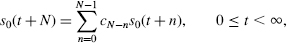## 8.4 THE CHARACTERISTIC POLYNOMIAL OF A LINEAR FEEDBACK SHIFT REGISTER

The characteristic polynomial of the N-stage LFSR with recursionand feedback coefficients {ci} isWe will always assume c0 = cN = 1.

Example 8.3

The LFSR with characteristic polynomial p(z) = 1 + z + z2 + z3 is shown in Figure 8.3. As p(z) does not divide 1 + zk for k = 1, 2, 3 and (1 + z)p(z) = 1 + z4, the exponent of p(z) is 4. Table 8.5 gives the output and states of this LFSR for three different initial states. Table 8.5 illustrates that the period of the sequence s0(0), s0(1), s0(2), … depends on the initial state s(0):

• s(0) = (0, 0, 1) produces a sequence of period 3,
• s(0) = (1, 0, 1) produces a sequence of period 2, and
• s(0) = (1, 1, 1) produces a sequence of period 1.

Proposition 8.3: [Beker and Piper, 1982, pp. 192–193] If p(z) = cN + cN−1z + ··· + c1zN−l + c0zN is the characteristic polynomial of the LFSR, then

 8.3a The period of the output sequence s0(0), s0(1), s0(2), … is a divisor of the exponent of p(z), and 8.3b If the initial state s(0) ≠ (0)N, the period of the output sequence s0(0), s0(1), s0(2), … is 2N − 1 if and only if p(z) is primitive.

Example 8.4

The LFSR with characteristic polynomial p(z) = 1 + z + z3 is shown in Figure 8.4. The exponent of p(z) was shown to be ...

Get Computer Security and Cryptography now with the O’Reilly learning platform.

O’Reilly members experience live online training, plus books, videos, and digital content from nearly 200 publishers.